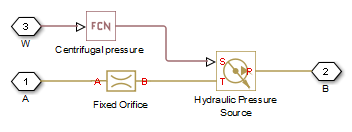# Rotating Pipe

Hydraulic pipeline created by bore in rotating housing

## Library

Pipelines

•## Description

The Rotating Pipe block models a hydraulic pipeline represented as a short bore drilled through a shaft at some angle to its axis, or as a short pipe that connects actuator or bearings, mounted on the shaft, with the channel inside the shaft. Such connections are typical for lubrication systems and for hydraulically-operated clutches and mechanisms. The shaft angular velocity is assumed to be constant or changing at very low speed. The block is implemented as a structural model, shown in the diagram below:The Fixed Orifice block simulates pressure losses in the bore. The pipe is assumed to be short enough to be characterized by a flow-pressure relationship similar to that of the fixed orifice. The model accounts for the laminar and turbulent flow regimes by monitoring the Reynolds number (`Re`) and comparing its value with the critical Reynolds number (`Recr`). The Hydraulic Pressure Source block represents pumping action developed by a rotating pipe, which is calculated with the equation:

`$p=\rho \cdot \frac{{\omega }^{2}\cdot {r}_{B}^{2}}{2}$`

where

 p Pressure at the pipe outlet ρ Fluid density ω Shaft angular velocity rB Radius of the pipe outlet

Connections A and B are conserving hydraulic ports associated with the pipe inlet and outlet, respectively. Connection W is the physical signal input port that provides the shaft angular velocity value.

## Basic Assumptions and Limitations

• The pipe is assumed to be short, to be simulated with the fixed orifice.

• Flow is assumed to be fully developed along the pipe length.

• The transition between laminar and turbulent regimes is assumed to be sharp and taking place exactly at `Re`=`Recr`.

• Fluid inertia is not taken into account.

• Fluid compressibility is not taken into account.

## Parameters

Pipe cross-section area

Cross-sectional area of the pipe. The default value is `1e-4` m^2.

Radius of rotation of the outer end of the pipe. The default value is `0.03` m.

Flow discharge coefficient

Semi-empirical parameter for pipe capacity characterization. Its value depends on the geometrical properties of the pipe, and usually is provided in textbooks or manufacturer data sheets. The default value is `0.6`.

Critical Reynolds number

The maximum Reynolds number for laminar flow. The transition from laminar to turbulent regime is assumed to take place when the Reynolds number reaches this value. The value of the parameter depends on the pipe geometrical profile. You can find recommendations on the parameter value in hydraulics textbooks. The default value is `12`, which corresponds to a round orifice in thin material with sharp edges.

## Global Parameters

Parameters determined by the type of working fluid:

• Fluid density

• Fluid kinematic viscosity

Use the Hydraulic Fluid block or the Custom Hydraulic Fluid block to specify the fluid properties.

## Examples

For an example of using the Rotating Pipe block to model a custom single-acting hydraulic cylinder, see the Centrifugal Force in Rotating Cylinder block reference page.

## Ports

The block has the following ports:

`A`

Hydraulic conserving port associated with the pipe inlet.

`B`

Hydraulic conserving port associated with the pipe outlet.

`W`

Physical signal port providing the angular velocity value.

## Version History

Introduced in R2010a